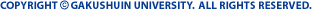Department of Mathematics Mathematics is the basis of science, and can even be described as an expression of human culture. In recent years, with the influence of mathematical science spreading beyond technology and into other fields, the importance of mathematics - the keystone and the origin of the discipline - has started to be widely recognized. Mastering the fundamentals of mathematics will ensure an ability to deal flexibly with the speed of scientific progress - indeed the age we live in actually demands the thorough study of this subject. At the Department of Mathematics, students start with a slow but sure look at the basics, the methodology and the philosophy of the discipline, whilst at the same time gaining understanding of mathematical science, including computer science. The department offers a well-balanced curriculum. For first and second year students, the core of the curriculum consists of classes in advanced calculus, linear algebra, set theory, elementary number theory, theory of ordinary differential equations and computer science. Each lecture is followed by an exercise class organized by one or more research associate, in which students are encouraged to find the joy of solving problems. Through practice in tackling carefully selected problems, students are able to perfect their understanding of basic concepts and go on to develop their ability to solve difficult problems. Even in the first year, students can work in seminars to discuss problems they are interested in. A variety of courses in contemporary mathematics are offered for third and fourth year students: theory of groups, modern abstract algebra, theory of numbers, algebraic geometry, general topology, algebraic topology, differential topology, differential geometry, theory of functions of a complex variable, measure theory, functional analysis, probability theory, numerical analysis, an introduction to computer programming, etc. Around one quarter of the students go on to the Graduate School or take up teaching at junior or high schools; the remainder enter a wide variety of businesses and industries, where they are able to find excellent positions.